# （3）小波变换原理及应用

## Part1-Introduction To The Wavelet Transform（简介）

1、Origin of the wavelet transform:
The theories of Wavelet originate from diffierent areas of study:

• Engineering

• Time-frequency analysis and Multiresolution Analysis

• Computer Vision

• Pyramidal algorithm

• Physics

• Pure Mathematics

2、Multiresolution Analysis（MRA-多分辨率分析与处理）

• Multiresolution analysis is about analyzing a signal based on the information appeared in different scales of such signal – to mimic human beings in analyzing signals.

• A signal of a certain “scale” refers to its best approximation at a certain resolution

• By “traveling” from the coarse scales toward the fine scales, one zooms in and arrives at a more exact representation of the given signal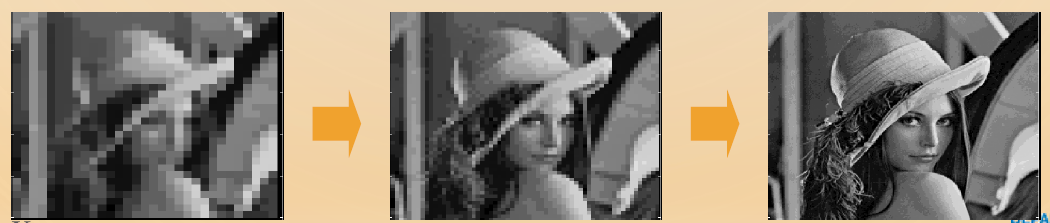3、The Discrete Wavelet Transform(离散小波变换)
A simple way to implement MAR is by using the Discrete Wavelet Transform(DWT):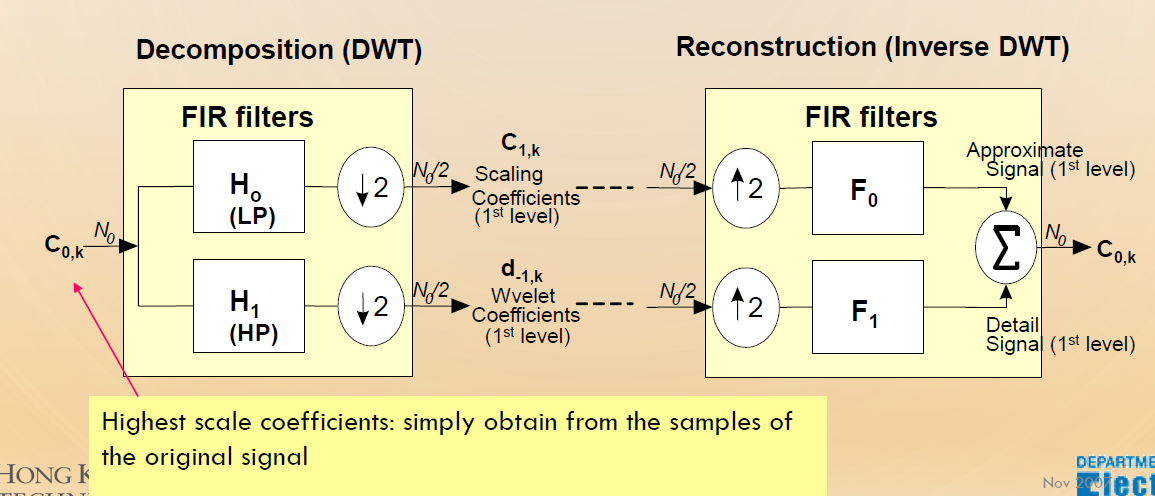The definition of wavelet transform shows that the wavelet analysis is a measure of similarity the basis functions(wavelets) and the original function .The coefficients ，named H0 for Low Pass Filter, and H1 for High Pass Filter, caculated indicate how close the function is to the danghter wavelet at that particular scale.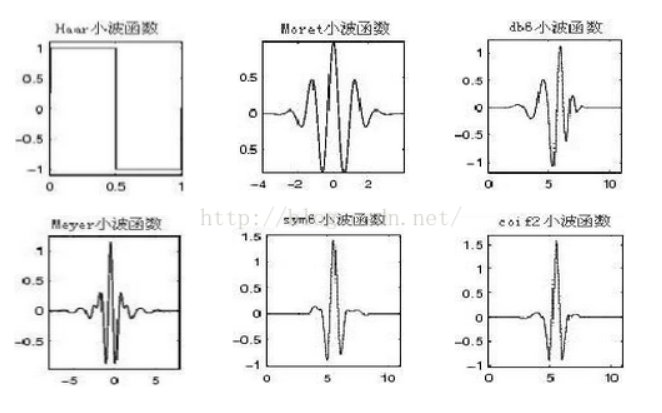## 1、一维Haar小波变换-分解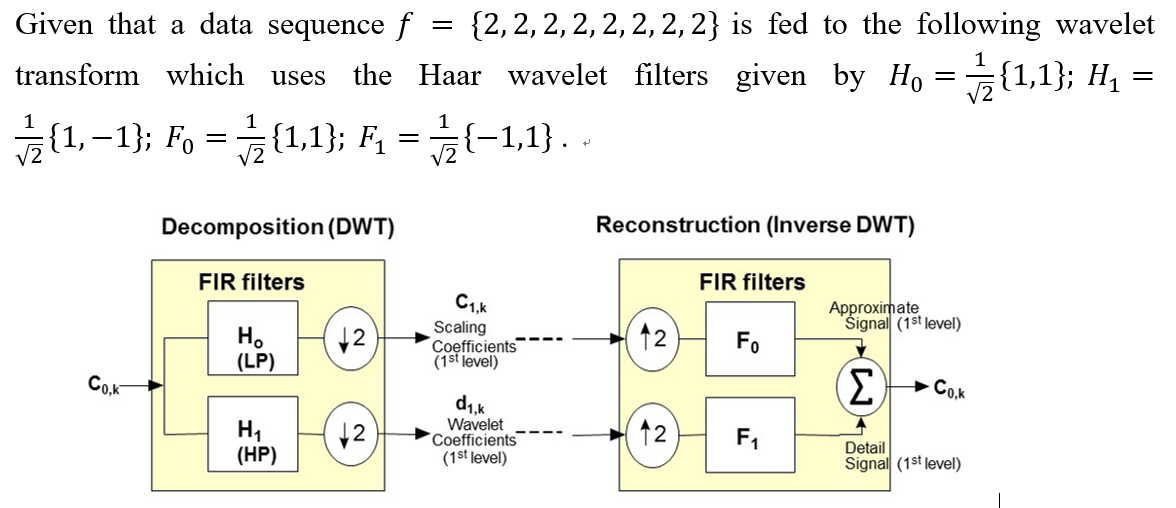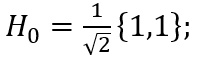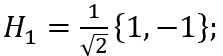“离散卷积”是两个离散序列x(n) 和h(n) 之间按照一定的规则将它们的有关序列值分别两两相乘再相加的一种特殊的运算。具体可用公式表示为: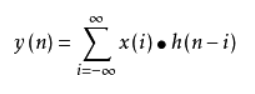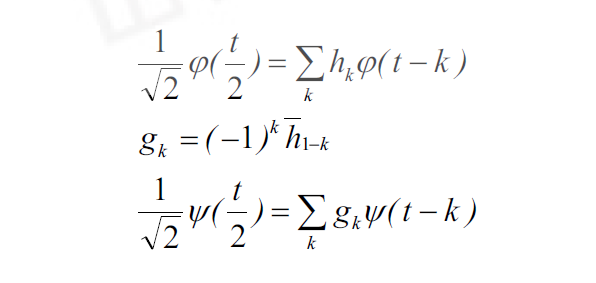## 3、二维Haar小波变换

(l)LL子带是由两个方向利用低通小波滤波器卷积后产生的小波系数，它是图像的近似表示。
(2)HL子带是在行方向利用低通小波滤波器卷积后，再用高通小波滤波器在列方向卷积而产生的小波系数，它表示图像的水平方向奇异特性。（水平子带）
(3)LH子带是在行方向利用高通小波滤波器卷积后，再用低通小波滤波器在列方向卷积而产生的小波系数，它表示图像的垂直方向奇异特性。（垂直子带）
(4)HH子带是由两个方向利用高通小波滤波器卷积后产生的小波系数，它表示图像的对角边缘特性。（对角子带）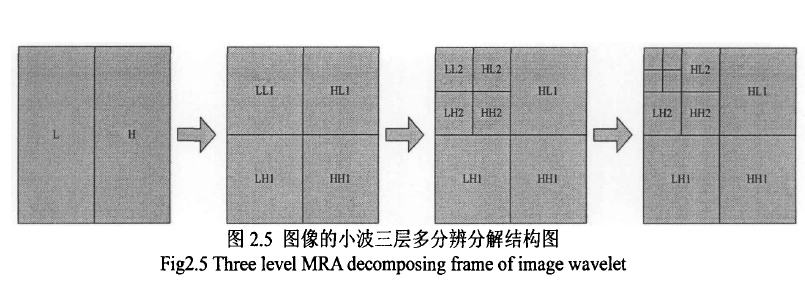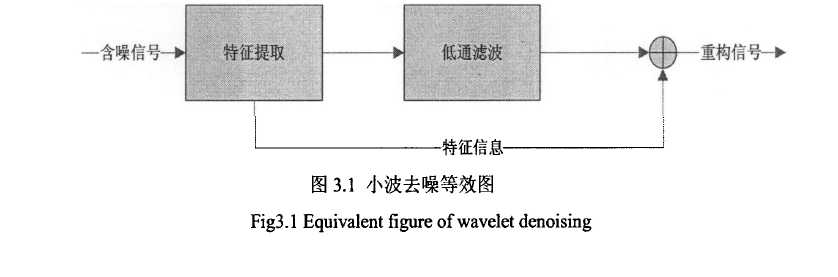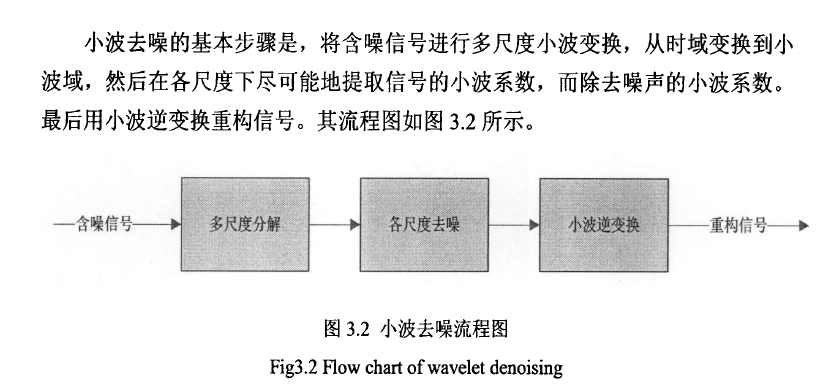## 4、小波阀值收缩去噪法:

1、 小波阀值去噪的基本思想:

Donoho提出的小波阀值去噪的基本思想是将信号通过小波变换（采用Mallat算法）后，信号产生的小波系数含有信号的重要信息，将信号经小波分解后小波系数较大，噪声的小波系数较小，并且噪声的小波系数要小于信号的小波系数，通过选取一个合适的阀值，大于阀值的小波系数被认为是有信号产生的，应予以保留，小于阀值的则认为是噪声产生的，置为零从而达到去噪的目的。其基本步骤为：
（1）分解：选定一种层数为N的小波对信号进行小波分解；
（2）阀值处理过程：分解后通过选取一合适的阀值，用阀值函数对各层系数进行量化；
（3）重构：用处理后的系数重构信号。

2、小波阀值去噪的基本问题

（1）小波基的选择：通常我们希望所选取的小波满足以下条件：正交性、高消失矩、紧支性、对称性或反对称性。但事实上具有上述性质的小波是不可能存在的，因为小波是对称或反对称的只有Haar小波，并且高消失矩与紧支性是一对矛盾，所以在应用的时候一般选取具有紧支的小波以及根据信号的特征来选取较为合适的小波。
（2）阀值的选择：直接影响去噪效果的一个重要因素就是阀值的选取，不同的阀值选取将有不同的去噪效果。目前主要有通用阀值（VisuShrink）、SureShrink阀值、Minimax阀值、BayesShrink阀值等。
（3）阀值函数的选择：阀值函数是修正小波系数的规则，不同的反之函数体现了不同的处理小波系数的策略。最常用的阀值函数有两种：一种是硬阀值函数，另一种是软阀值函数。还有一种介于软、硬阀值函数之间的Garrote函数。

## 实例：基于通用阈值和SURE阈值的小波去噪方法的MATLAB实现

%
% 2d wavelet shrinkage image denoising with universal threshold
%
close all

% Load image
imagefilename = 'Lena512Gray.bmp';
thewavelet = 'db4';
noise_var = 300;

x = double(imread(imagefilename));
n = sqrt(noise_var)*randn(size(x));
xn = x + n;

% Make sure the additive noise will not make the signal component to have
% magnitude larger than 255.
for i=1:size(x,1)
for j=1:size(x,2)
if (xn(i,j) > 255)
xn(i,j) = 255;
end
end
end

std_noise = sqrt(sum(sum((xn-x).^2))/length(x)^2);

figure
imagesc([x,xn]);
axis image
colormap(gray);
title('original, noisy');

% 2d wavelet transform
% 1 levels decomposition with periodic extension.
dwtmode('per');
[ca1,ch1,cv1,cd1] = dwt2(x,  thewavelet);
[na1,nh1,nv1,nd1] = dwt2(xn, thewavelet);
[na2,nh2,nv2,nd2] = dwt2(na1, thewavelet);

% Display
figure
imagesc([ca1,ch1;cv1,cd1]);
axis image
colormap(gray);
title('1st level decomposition with periodic extension.');
figure
imagesc([na1,nh1;nv1,nd1]);
axis image
colormap(gray);
title('1st level decomposition with periodic extension of noisy image.');

% First normalize the wavelet coefficients such that the noise component
% has a variance of 1
nor_nh1 = nh1/std_noise;
nor_nv1 = nv1/std_noise;
nor_nd1 = nd1/std_noise;

% Find the threshold!
disp('Universal threshold used for level 1')
thr_uni = zeros(1,3);
thr_uni(:) = sqrt(2*log(size(x,1)*size(x,2)));

% Shrinkage (SURE)

thr_sure = zeros(1,3);
thr_sure(1) = thselect(nor_nh1,'rigrsure');
thr_sure(2) = thselect(nor_nv1,'rigrsure');
thr_sure(3) = thselect(nor_nd1,'rigrsure');

disp('SURE threshold used for level 1');

% Shrinkage (universal threshold)
dnh1_uni = wthresh(nor_nh1,'s',thr_uni(1));
dnv1_uni = wthresh(nor_nv1,'s',thr_uni(2));
dnd1_uni = wthresh(nor_nd1,'s',thr_uni(3));
% Shrinkage (SURE)
dnh1_sure = wthresh(nor_nh1,'s',thr_sure(1));
dnv1_sure = wthresh(nor_nv1,'s',thr_sure(2));
dnd1_sure = wthresh(nor_nd1,'s',thr_sure(3));

% Denormalize the denoised wavelet coefficients
dnh1_uni = dnh1_uni*std_noise;
dnv1_uni = dnv1_uni*std_noise;
dnd1_uni = dnd1_uni*std_noise;

dnh1_sure = dnh1_sure*std_noise;
dnv1_sure = dnv1_sure*std_noise;
dnd1_sure = dnd1_sure*std_noise;

% Reconstruction (universal threshold)
rx = idwt2(ca1,ch1,cv1,cd1,thewavelet,size(x));
rdnx_uni = idwt2(na1,dnh1_uni,dnv1_uni,dnd1_uni,thewavelet,size(x));

% Reconstruction (SURE)
rdnx_sure = idwt2(na1,dnh1_sure,dnv1_sure,dnd1_sure,thewavelet,size(x));

figure
imagesc([x,xn,rdnx_uni,rdnx_sure]);
axis image
colormap(gray);
title('original, noisy, denoised(universal), denoised(SURE)');

res = sprintf('SNR of noisy image: %f dB', snr(x, xn));
res1 = sprintf('SNR of denoised image (universal threshold): %f dB', snr(x, rdnx_uni));
res2 = sprintf('SNR of denoised image (SURE): %f dB', snr(x, rdnx_sure));
disp(res);
disp(res1);
disp(res2);Fig.1 Lena 原图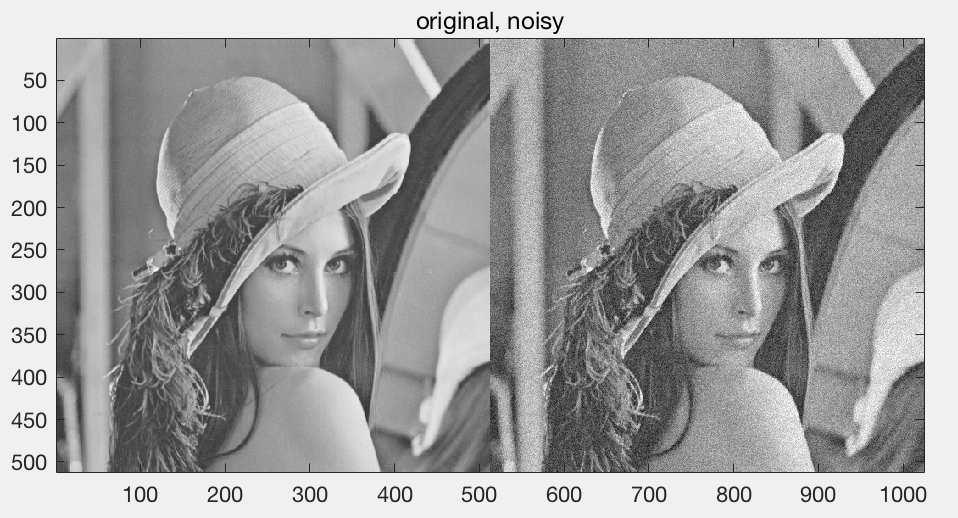Fig .2 加入噪声之后的图像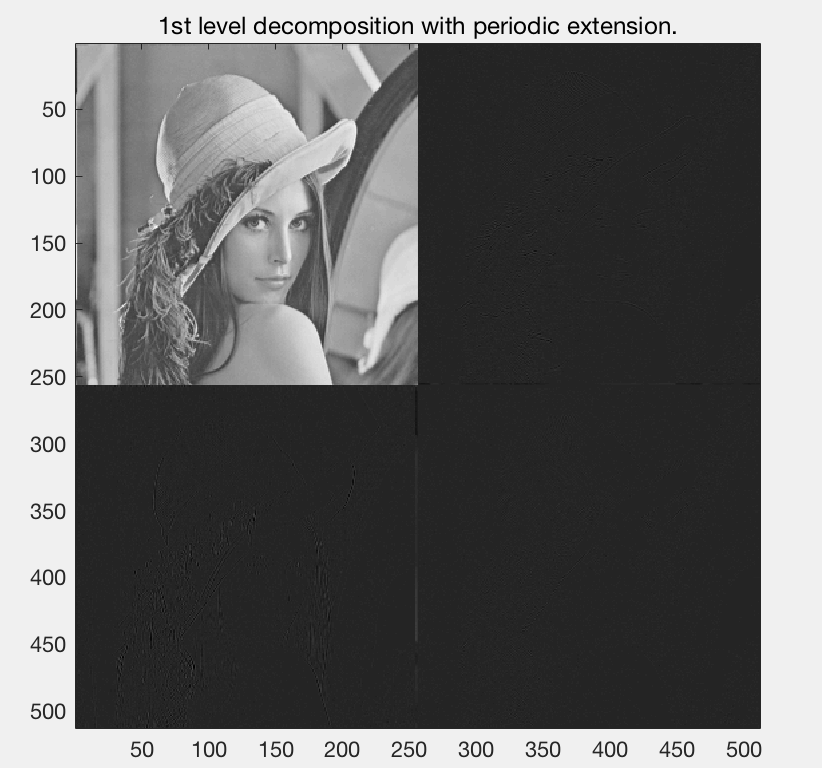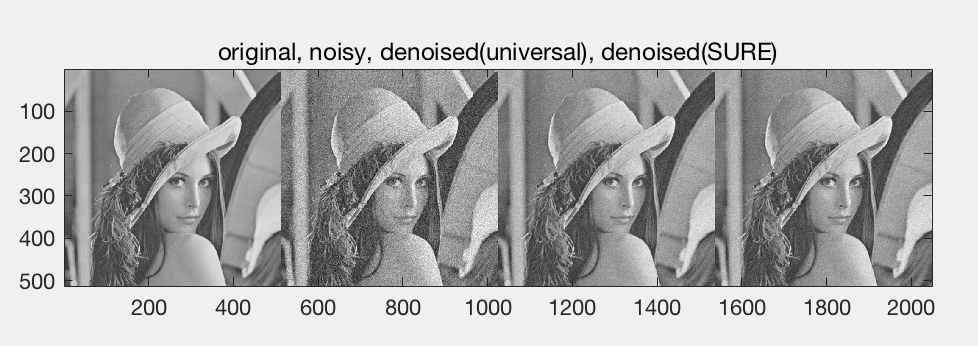SNR of noisy image: 38.468755 dB
SNR of denoised image (universal threshold): 40.259733 dB
SNR of denoised image (SURE): 41.010018 dB